Log Service provides the following types of date and time functions that you can use to analyze log data: time function, date function, truncation function, interval function, and time series padding function. You can use the functions to convert the date and time formats of log data. You can also use the functions to group and aggregate log data. This topic describes the syntax of date and time functions. This topic also provides examples on how to use the functions.

Notice
• The timestamp of a log entry in Log Service is accurate to the second. Therefore, you can specify the time format only accurate to the second. For more information, see Formats.
• You need to specify the time format only for the time in a time string. Other parameters such as the time zone are not required. For more information, see Formats.
• Each log entry in Log Service contains the reserved __time__ field. The value of the field is a UNIX timestamp. For example, 1592374067 indicates 2020-06-17 14:07:47.
• If you want to use strings in analytic statements, you must enclose strings in single quotation marks (''). Strings that are not enclosed or enclosed in double quotation marks ("") indicate field names or column names. For example, 'status' indicates the status string, and status or "status" indicates the status log field.
Type Function Syntax Description
Date functions current_date function current_date Returns the current date.
current_time function current_time Returns the current time.
current_timestamp function current_timestamp Returns the current date and time.
current_timezone function current_timezone() Returns the current time zone.
from_iso8601_date function from_iso8601_date(x) Converts an ISO 8601-formatted date expression to a date expression.
from_iso8601_timestamp function from_iso8601_timestamp(x) Converts an ISO 8601-formatted datetime expression to a timestamp expression that contains a time zone.
from_unixtime function from_unixtime(x) Converts a UNIX timestamp to a timestamp expression.
from_unixtime(x,time zone) Converts a UNIX timestamp to a timestamp expression that contains a time zone.
localtime function localtime Returns the local time.
localtimestamp function localtimestamp Returns the local date and time.
now function now() Returns the current date and time. This function is equivalent to the current_timestamp function.
to_unixtime function to_unixtime(x) Converts a timestamp expression to a UNIX timestamp.
Time functions date_format function date_format(x,format) Converts a timestamp expression to a datetime expression in a specified format.
date_parse function date_parse(x,format) Represents a datetime string in a specified format, and then converts the datetime string to a timestamp expression.
Truncation function date_trunc function date_trunc('unit',x) Truncates a datetime expression based on the specified part of a point in time. You can use the date_trunc function to truncate a point in time by millisecond, second, minute, hour, day, month, or year.
date_diff function date_diff(unit, x, y) Returns the time difference between two time expressions. For example, you can calculate the difference between x and the y by unit.
Time series padding function time_series function time_series(x, window, format, padding_data) Adds the missing data in the specified time window.

## current_date function

The current_date function is used to return the current date. The return value is in the YYYY-MM-DD format.

### Syntax

``current_date``

The date type.

### Examples

Query the logs of the previous day.

• Query statement
``````* |
SELECT
*
FROM  log
WHERE
__time__ < to_unixtime(current_date)
AND __time__ > to_unixtime(date_add('day', -1, current_date))``````
• Query and analysis result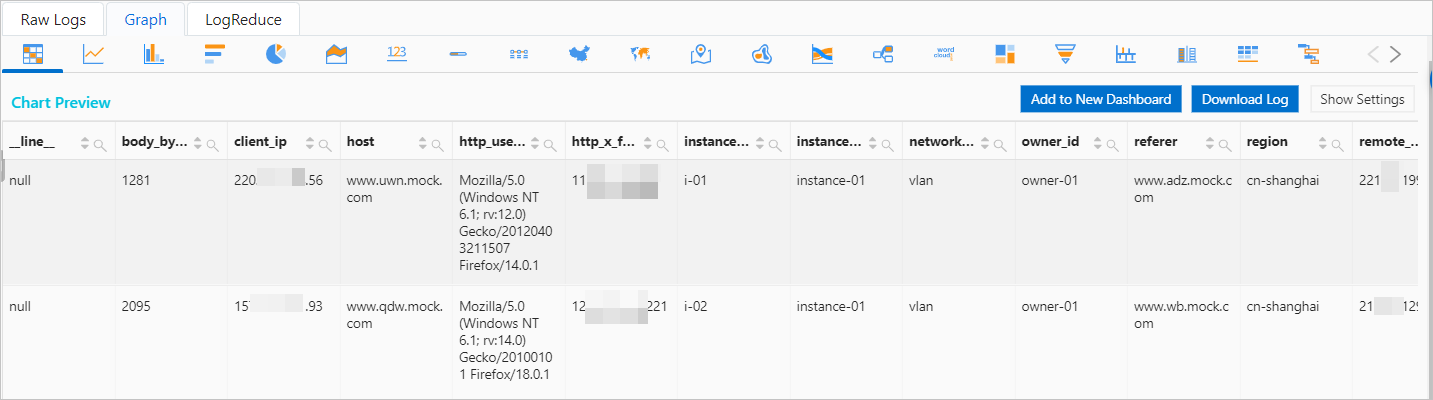## current_time function

The current_time function is used to query the current time and time zone. The return value is in the HH:MM:SS.Ms Time_zone format.

### Syntax

``current_time``

The time type.

### Examples

Query the current time and time zone.

• Query statement
``* | select current_time``
• Query and analysis result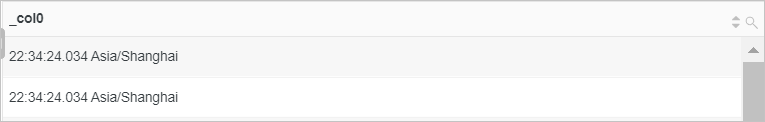## current_timestamp function

The current_timestamp function is used to query the current date, time, and time zone. The return value is in the YYYY-MM-DD HH:MM:SS.Ms Time_zone format.

### Syntax

``current_timestamp``

### Return value type

The timestamp type.

### Examples

Query the logs of the previous day.

• Query statement
``````* |
SELECT
*
FROM  log
WHERE
__time__ < to_unixtime(current_timestamp)
AND __time__ > to_unixtime(date_add('day', -1, current_timestamp))``````
• Query and analysis result## current_timezone function

The current_timezone function is used to return the current time zone.

### Syntax

``current_timezone()``

### Return value type

The varchar type.

### Examples

Query the current time zone.

• Query statement
``* | select current_timezone()``
• Query and analysis result## from_iso8601_date function

The from_iso8601_date function is used to convert an ISO 8601-formatted date expression to a date expression. The return value is in the YYYY-MM-DD format.

### Syntax

``from_iso8601_date(x)``

### Parameters

Parameter Description
x The value of this parameter is an ISO 8601-formatted date expression.

The date type.

### Examples

Convert the value of the time field to a date expression.

• Sample field
``time:2020-05-03``
• Query statement
``* | select from_iso8601_date(time)``
• Query and analysis result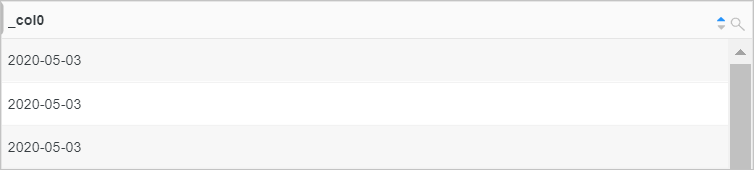## from_iso8601_timestamp function

The from_iso8601_timestamp function is used to convert an ISO 8601-formatted datetime expression to a timestamp expression. The return value is in the YYYY-MM-DD HH:MM:SS.Ms Time_zone format.

### Syntax

``from_iso8601_timestamp(x)``

### Parameters

Parameter Description
x The value of this parameter is an ISO 8601-formatted datetime expression.

### Return value type

The timestamp type.

### Examples

Convert the value of the time field to a timestamp expression.

• Sample field
``time:2020-05-03T17:30:08``
• Query statement
``* | select from_iso8601_timestamp(time)``
• Query and analysis result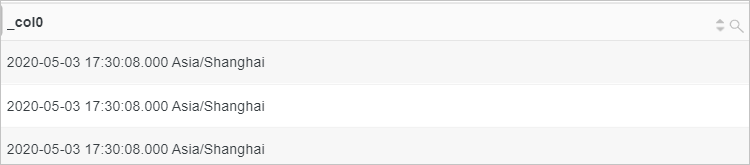## from_unixtime function

The from_unixtime function is used to convert a UNIX timestamp to a timestamp expression. The return value is in the YYYY-MM-DD HH:MM:SS.Ms or YYYY-MM-DD HH:MM:SS.Ms Time_zone format.

### Syntax

• To convert a UNIX timestamp to a timestamp expression that does not contain a time zone, use the following syntax:
``from_unixtime(x)``
• To convert a UNIX timestamp to a timestamp expression that contains a time zone, use the following syntax:
``from_unixtime(x,time zone)``

### Parameters

Parameter Description
x The value of this parameter is a UNIX timestamp.
time zone The time zone, for example, Asia/Shanghai.

### Return value type

The timestamp type.

### Examples

Convert the value of the time field to a timestamp expression that contains a time zone.

• Sample field
``time:1626774758``
• Query statement
``* | select from_unixtime(time,'Asia/shanghai')``
• Query and analysis result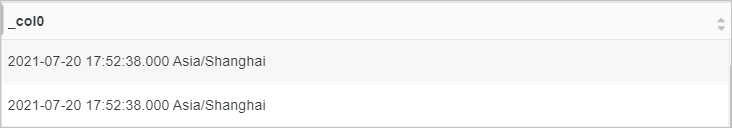## localtime function

The localtime function is used to return the local time. The return value is in the HH:MM:SS.Ms format.

### Syntax

``* | select localtime``

The time type.

### Examples

Query the local time.

• Query statement
``* | select localtime``
• Query and analysis result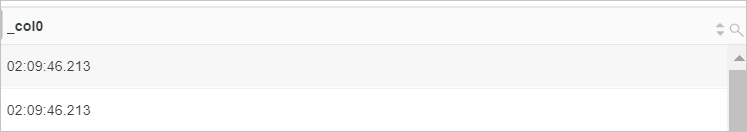## localtimestamp function

The localtimestamp function is used to return the local date and time. The return value is in the YYYY-MM-DD HH:MM:SS.Ms Time_zone format.

### Syntax

``localtimestamp``

### Return value type

The timestamp type.

### Examples

Query the logs of the previous day.

• Query statement
``````* |
SELECT
*
FROM  log
WHERE
__time__ < to_unixtime(localtimestamp)
AND __time__ > to_unixtime(date_add('day', -1, localtimestamp))``````
• Query and analysis result## now function

The now function is used to return the current date and time. The return value is in the YYYY-MM-DD HH:MM:SS.Ms Time_zone format. This function is equivalent to the current_timestamp function.

### Syntax

``now()``

### Return value type

The timestamp type.

### Examples

Query the logs of the previous day.

• Query statement
``````* |
SELECT
*
FROM  log
WHERE
__time__ < to_unixtime(now())
AND __time__ > to_unixtime(date_add('day', -1, now()))``````
• Query and analysis result## to_unixtime function

The to_unixtime function is used to convert a timestamp expression to a UNIX timestamp.

### Syntax

``to_unixtime(x)``

### Parameters

Parameter Description
x The value of this parameter is a timestamp expression.

The double type.

### Examples

Query the logs of the previous day.

• Query statement
``````* |
SELECT
*
FROM  log
WHERE
__time__ < to_unixtime(now())
AND __time__ > to_unixtime(date_add('day', -1, now()))``````
• Query and analysis result## date_format function

The date_format function is used to convert a timestamp expression to a datetime expression in a specified format.

### Syntax

``date_format(x,format)``

### Parameters

Parameter Description
x The value of this parameter is a timestamp expression.
format The format of the datetime expression to which you want to convert the timestamp expression. For more information, see Formats.

### Return value type

The varchar type.

### Examples

Query NGINX request statuses, calculate the number of NGINX requests, and then display the query and analysis result in chronological order. To do this, use the date_trunc function to truncate the log time by minute and use the date_format function to convert the time to the `%H:%i` format. Then, calculate the number of requests for each status code per minute and display the query and analysis result on a flow chart.

• Query statement
``````* |
SELECT
date_format(date_trunc('minute', __time__), '%H:%i') AS time,
COUNT(1) AS count,
status
GROUP BY
time,
status
ORDER BY
time``````
• Query and analysis result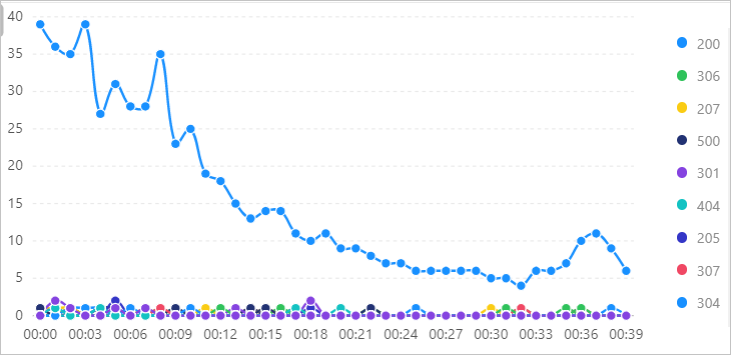## date_parse function

The date_parse function is used to convert a datetime string to a timestamp expression in a specified format.

### Syntax

``date_parse(x,format)``

### Parameters

Parameter Description
x The value of this parameter is a datetime string.
format The format of the datetime expression to which you want to convert the datetime string. For more information, see Formats.

### Return value type

The timestamp type.

### Examples

Convert the values of the StartTime and EndTime fields to timestamp expressions and calculate the time difference between the two timestamp expressions.

• Query statement
``````*|
SELECT
date_parse(StartTime, '%Y-%m-%d %H:%i') AS "StartTime",
date_parse(EndTime, '%Y-%m-%d %H:%i') AS "EndTime",
date_diff('hour', StartTime, EndTime) AS "Time Difference (Hours)"``````
• Query and analysis result## date_trunc function

The date_trunc function is used to truncate a datetime expression based on the specified part of a point in time. You can use the date_trunc function to truncate a point in time by millisecond, second, minute, hour, day, month, or year. This function is suitable for time-based statistics.

### Syntax

``date_trunc(unit,x)``

### Parameters

Parameter Description
unit The unit of time. Valid values: millisecond, second, minute, hour, day, week, month, quarter, and year. For more information, see Units.
x The value of this parameter is a datetime expression.
Note You can use the date_trunc function to truncate a point in time only based on a fixed interval, such as 1 minute or 1 hour. To truncate a point in time based on a specified interval such as 5 minutes, you must use a GROUP BY clause based on the modulus method.
``* | SELECT count(1) AS pv,  __time__ - __time__ %300 AS time GROUP BY time LIMIT 100``

### Return value type

The type of the return value is the same as the type of the datetime expression that you specify.

### Examples

Calculate the average request durations by minute, and then group and sort the average request durations by time.

• Query statement
``````* |
SELECT
date_trunc('minute', __time__) AS time,
truncate (avg(request_time)) AS avg_time,
current_date AS date
GROUP BY
time
ORDER BY
time DESC
LIMIT
100``````
• Query and analysis result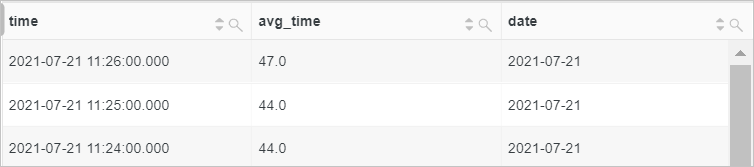The date_add function is used to add a specified interval to or subtract a specified interval from a date or time.

### Syntax

``date_add(unit, n,x)``

### Parameters

Parameter Description
unit The unit of time. Valid values: millisecond, second, minute, hour, day, week, month, quarter, and year. For more information, see Units.
n The interval.
x The value of this parameter is a timestamp expression.

### Return value type

The timestamp type.

### Examples

Query the logs of the previous day.

• Query statement
``````* |
SELECT
*
FROM  log
WHERE
__time__ < to_unixtime(current_date)
AND __time__ > to_unixtime(date_add('day', -1, current_date))``````
• Query and analysis result## date_diff function

The date_diff function is used to calculate the time difference between two dates or points in time.

### Syntax

``date_diff(unit, x, y)``

### Parameters

Parameter Description
unit The unit of time. Valid values: millisecond, second, minute, hour, day, week, month, quarter, and year. For more information, see Units.
x The value of this parameter is a timestamp expression.
y The value of this parameter is a timestamp expression.

The bigint type.

### Examples

Calculate the usage duration of a server based on the UsageStartTime and UsageEndTime fields.

• Query statement
``* | SELECT  date_diff('hour', UsageStartTime, UsageEndTime) AS "Time Difference (Hours)"``
• Query and analysis result## time_series function

The time_series function is used to add the missing data in the specified time window.
Notice You must use the time_series function together with the GROUP BY and ORDER BY clauses. You cannot use the DESC keyword in the ORDER BY clause to sort data returned in descending order.

### Syntax

``time_series(x, window_time, format, padding_data)``

### Parameters

Parameter Description
x The sequence of time, for example, __time__. The value of this parameter is of the long type or the timestamp type.
window_time The size of the window. Valid units: s (seconds), m (minutes), h (hours), and d (days). For example, you can set the window parameter to 2h, 5m, or 3d.
format The format in which you want the function to return the value. For more information, see Formats.
• last: adds the value of the last point in time.
• next: adds the value of the next point in time.
• avg: adds the average value of the last and next values.

The bigint type.

### Examples

Add missing data with 0 by 2 hours.

• Query statement
``* | select time_series(__time__, '2h', '%Y-%m-%d %H:%i:%s', '0')  as time, count(*) as num from log group by time order by time                        ``
• Query and analysis result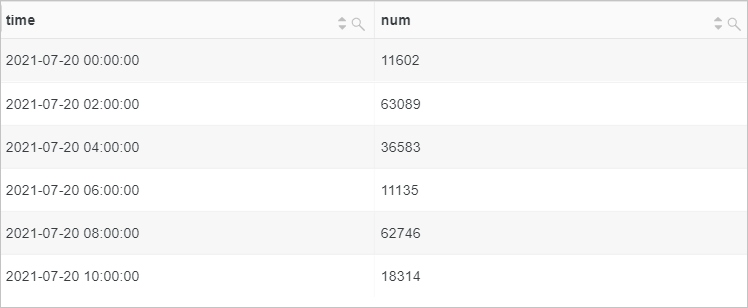## References

• Formats
format Description
%a The abbreviated day name, for example, Sun or Sat.
%b The abbreviated month name, for example, Jan or Dec.
%c The month. Valid values: 1 to 12.
%D The day of the month, for example, 0th, 1st, 2nd, or 3rd.
%d The day of the month. Valid values: 01 to 31.
%e The day of the month. Valid values: 1 to 31.
%H The hour in the 24-hour clock.
%h The hour in the 12-hour clock.
%I The hour in the 12-hour clock.
%i The minutes. Valid values: 00 to 59.
%j The day of the year. Valid values: 001 to 366.
%k The hours. Valid values: 0 to 23.
%l The hours. Valid values: 1 to 12.
%M The full month name, for example, January or December.
%m The month. Valid values: 01 to 12.
%p The abbreviation that indicates the morning or afternoon. Valid values: AM and PM.
%r The time in the 12-hour clock. The time is in the `hh:mm:ss AM/PM` format.
%S The seconds. Valid values: 00 to 59.
%s The seconds. Valid values: 00 to 59.
%T The time in the 24-hour clock. The time is in the `hh:mm:ss` format.
%V The week number of the year. Sunday is the first day of each week. Valid values: 01 to 53.
%v The week number of the year. Monday is the first day of each week. Valid values: 01 to 53.
%W The full day name, for example, Sunday or Saturday.
%w The day of the week as a number. The value 0 indicates Sunday.
%Y The four-digit year number, for example, 2020.
%y The two-digit year number, for example, 20.
%% Escapes the percent sign (%).
• Units
unit Description
millisecond Unit: milliseconds.
second Unit: seconds.
minute Unit: minutes.
hour Unit: hours.
day Unit: days.
week Unit: weeks.
month Unit: months.
quarter Unit: quarters.
year Unit: years.